Anúncio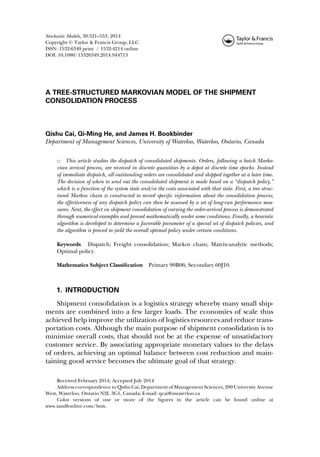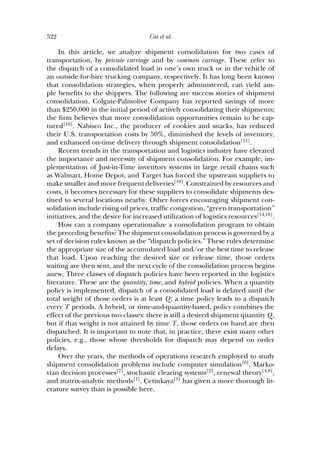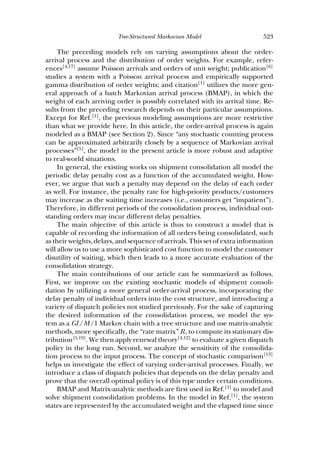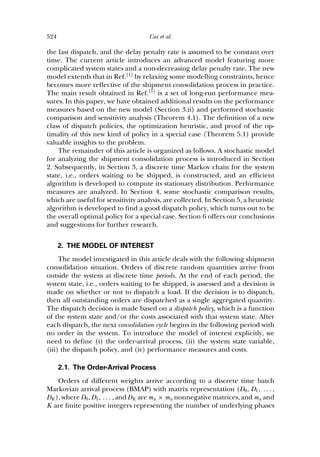Anúncio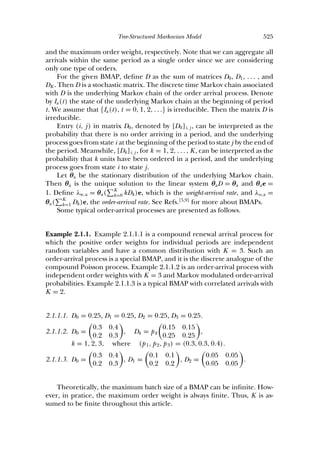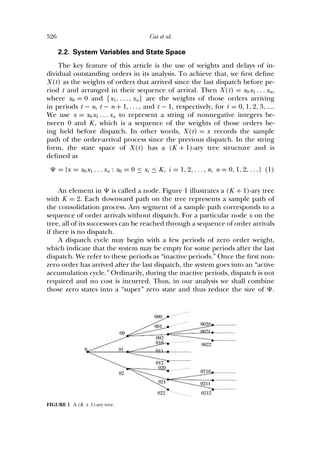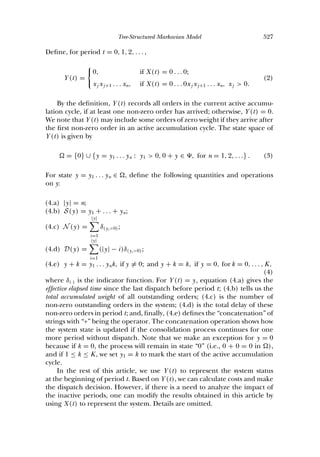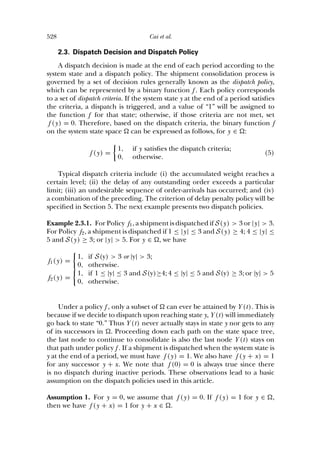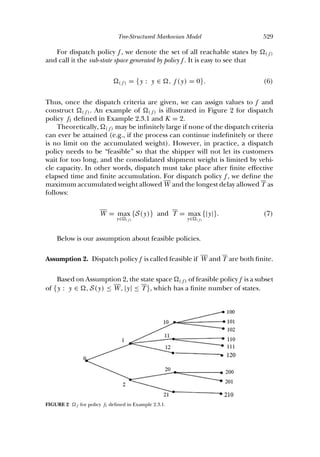Anúncio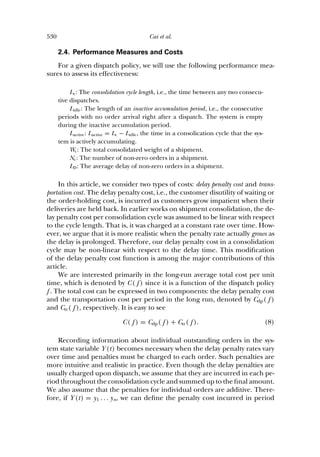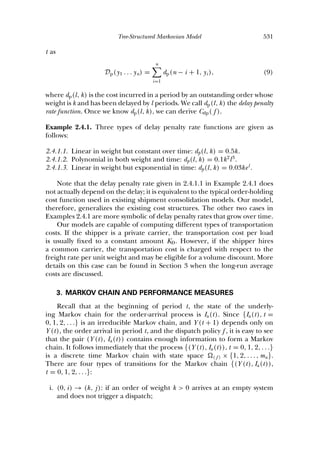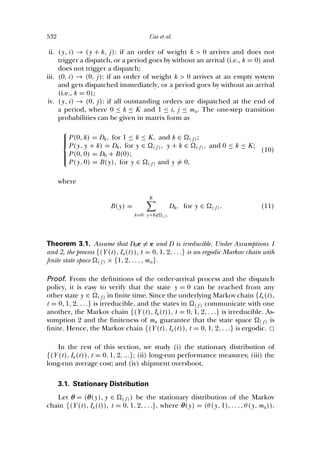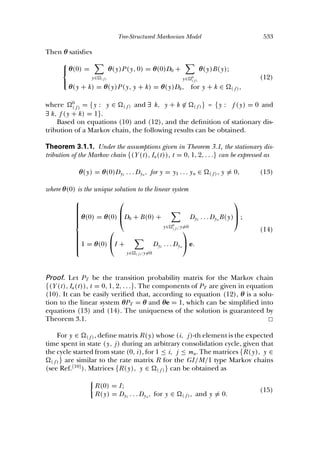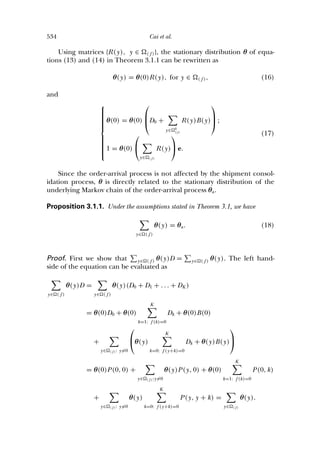Anúncio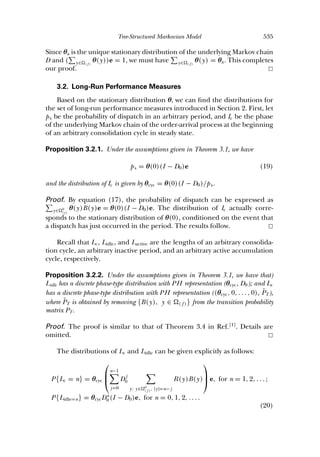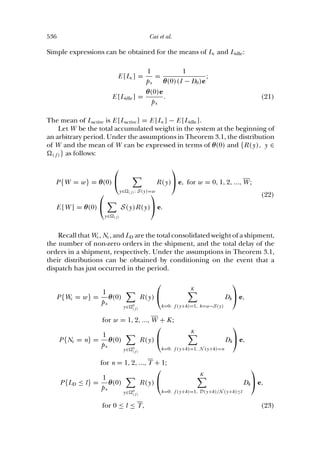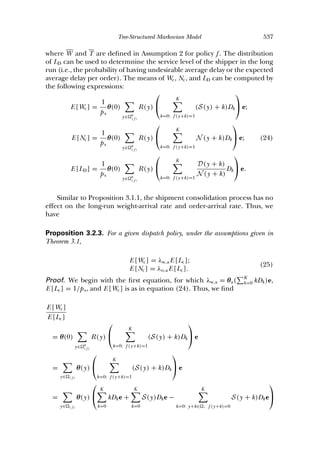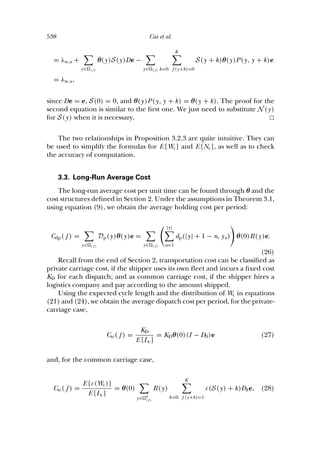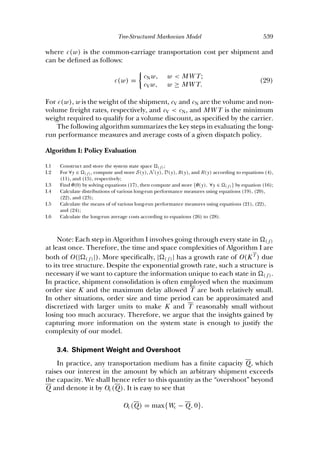Anúncio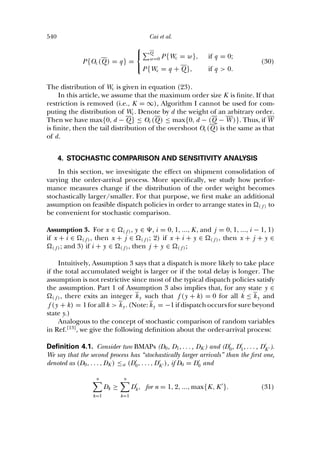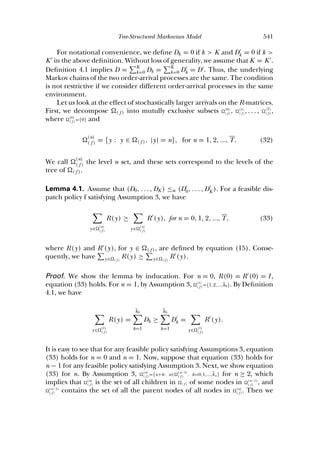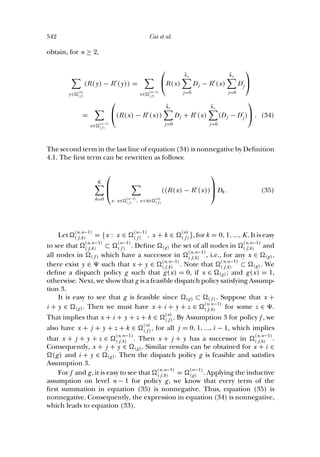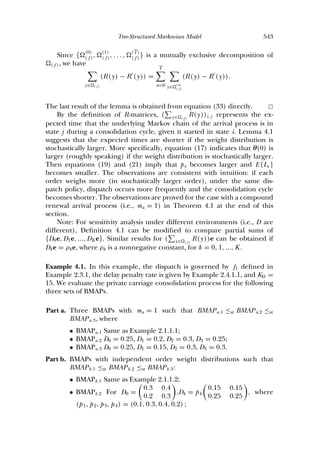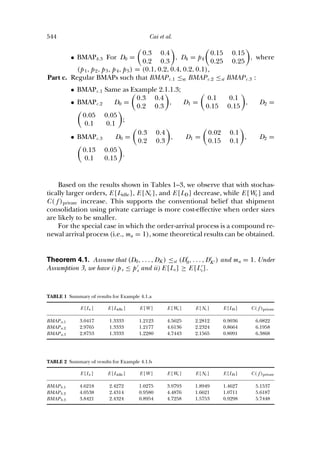Anúncio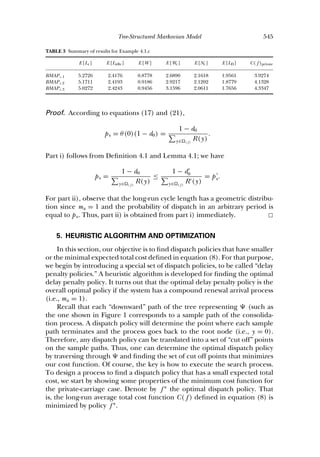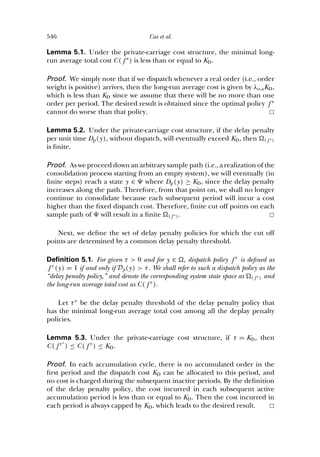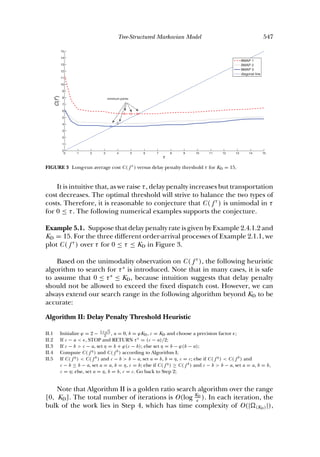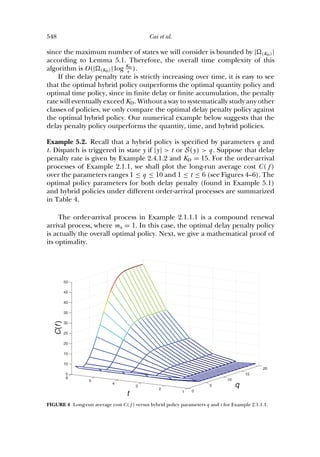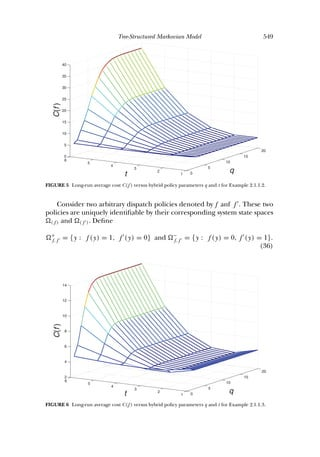Anúncio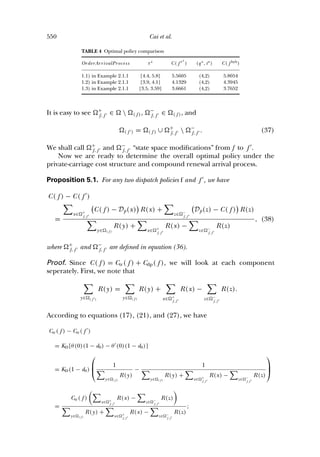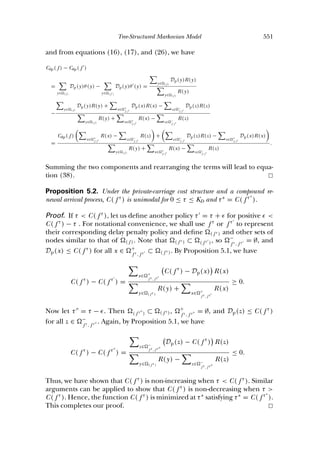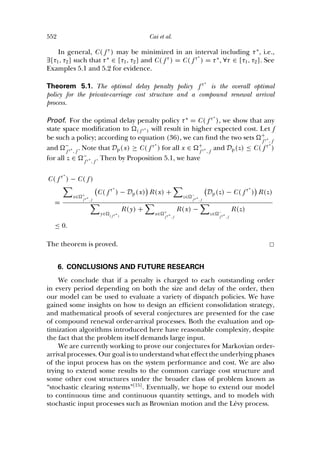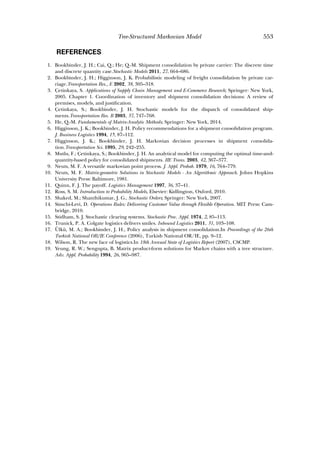Próximos SlideShares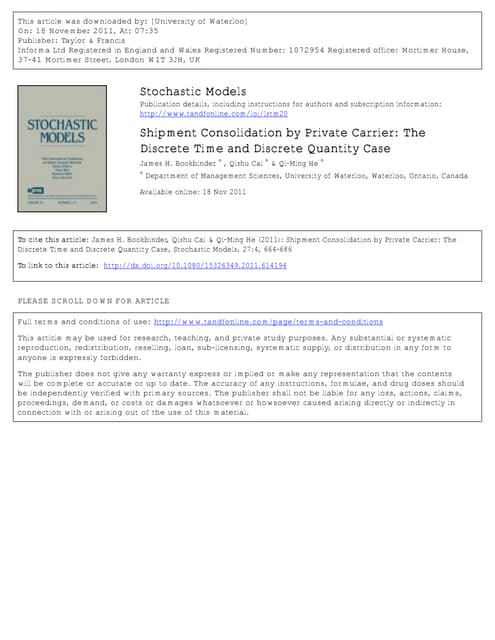2011_SM_Bookbinder, He and Cai
Carregando em ... 3
1 de 33
Anúncio

### Cai et al, 2014

2. 522 Cai et al. In this article, we analyze shipment consolidation for two cases of transportation, by private carriage and by common carriage. These refer to the dispatch of a consolidated load in one’s own truck or in the vehicle of an outside for-hire trucking company, respectively. It has long been known that consolidation strategies, when properly administered, can yield am- ple beneﬁts to the shippers. The following are success stories of shipment consolidation. Colgate-Palmolive Company has reported savings of more than \$250,000 in the initial period of actively consolidating their shipments; the ﬁrm believes that more consolidation opportunities remain to be cap- tured . Nabisco Inc., the producer of cookies and snacks, has reduced their U.S. transportation costs by 50%, diminished the levels of inventory, and enhanced on-time delivery through shipment consolidation . Recent trends in the transportation and logistics industry have elevated the importance and necessity of shipment consolidation. For example, im- plementation of Just-in-Time inventory systems in large retail chains such as Walmart, Home Depot, and Target has forced the upstream suppliers to make smaller and more frequent deliveries . Constrained by resources and costs, it becomes necessary for these suppliers to consolidate shipments des- tined to several locations nearby. Other forces encouraging shipment con- solidation include rising oil prices, trafﬁc congestion, “green transportation” initiatives, and the desire for increased utilization of logistics resources[14,18] . How can a company operationalize a consolidation program to obtain the preceding beneﬁts? The shipment consolidation process is governed by a set of decision rules known as the “dispatch policies.” These rules determine the appropriate size of the accumulated load and/or the best time to release that load. Upon reaching the desired size or release time, those orders waiting are then sent, and the next cycle of the consolidation process begins anew. Three classes of dispatch policies have been reported in the logistics literature. These are the quantity, time, and hybrid policies. When a quantity policy is implemented, dispatch of a consolidated load is delayed until the total weight of those orders is at least Q; a time policy leads to a dispatch every T periods. A hybrid, or time-and-quantity-based, policy combines the effect of the previous two classes: there is still a desired shipment quantity Q, but if that weight is not attained by time T, those orders on hand are then dispatched. It is important to note that, in practice, there exist many other policies, e.g., those whose thresholds for dispatch may depend on order delays. Over the years, the methods of operations research employed to study shipment consolidation problems include computer simulation , Marko- vian decision processes , stochastic clearing systems , renewal theory[4,8] , and matrix-analytic methods . C¸ etinkaya has given a more thorough lit- erature survey than is possible here.
3. Tree-Structured Markovian Model 523 The preceding models rely on varying assumptions about the order- arrival process and the distribution of order weights. For example, refer- ences[4,17] assume Poisson arrivals and orders of unit weight; publication studies a system with a Poisson arrival process and empirically supported gamma distribution of order weights; and citation utilizes the more gen- eral approach of a batch Markovian arrival process (BMAP), in which the weight of each arriving order is possibly correlated with its arrival time. Re- sults from the preceding research depends on their particular assumptions. Except for Ref. , the previous modeling assumptions are more restrictive than what we provide here. In this article, the order-arrival process is again modeled as a BMAP (see Section 2). Since “any stochastic counting process can be approximated arbitrarily closely by a sequence of Markovian arrival processes” , the model in the present article is more robust and adaptive to real-world situations. In general, the existing works on shipment consolidation all model the periodic delay penalty cost as a function of the accumulated weight. How- ever, we argue that such a penalty may depend on the delay of each order as well. For instance, the penalty rate for high-priority products/customers may increase as the waiting time increases (i.e., customers get “impatient”). Therefore, in different periods of the consolidation process, individual out- standing orders may incur different delay penalties. The main objective of this article is thus to construct a model that is capable of recording the information of all orders being consolidated, such as their weights, delays, and sequence of arrivals. This set of extra information will allow us to use a more sophisticated cost function to model the customer disutility of waiting, which then leads to a more accurate evaluation of the consolidation strategy. The main contributions of our article can be summarized as follows. First, we improve on the existing stochastic models of shipment consoli- dation by utilizing a more general order-arrival process, incorporating the delay penalty of individual orders into the cost structure, and introducing a variety of dispatch policies not studied previously. For the sake of capturing the desired information of the consolidation process, we model the sys- tem as a GI/M/1 Markov chain with a tree structure and use matrix-analytic methods, more speciﬁcally, the “rate matrix” R, to compute its stationary dis- tribution[5,19] . We then apply renewal theory[4,12] to evaluate a given dispatch policy in the long run. Second, we analyze the sensitivity of the consolida- tion process to the input process. The concept of stochastic comparison helps us investigate the effect of varying order-arrival processes. Finally, we introduce a class of dispatch policies that depends on the delay penalty and prove that the overall optimal policy is of this type under certain conditions. BMAP and Matrix-analytic methods are ﬁrst used in Ref. to model and solve shipment consolidation problems. In the model in Ref. , the system states are represented by the accumulated weight and the elapsed time since
5. Tree-Structured Markovian Model 525 and the maximum order weight, respectively. Note that we can aggregate all arrivals within the same period as a single order since we are considering only one type of orders. For the given BMAP, deﬁne D as the sum of matrices D0, D1, . . . , and DK . Then D is a stochastic matrix. The discrete time Markov chain associated with D is the underlying Markov chain of the order arrival process. Denote by Ia(t) the state of the underlying Markov chain at the beginning of period t. We assume that {Ia(t), t = 0, 1, 2, . . .} is irreducible. Then the matrix D is irreducible. Entry (i, j) in matrix D0, denoted by [D0]i, j , can be interpreted as the probability that there is no order arriving in a period, and the underlying process goes from state i at the beginning of the period to state j by the end of the period. Meanwhile, [Dk]i, j , for k = 1, 2, . . . , K, can be interpreted as the probability that k units have been ordered in a period, and the underlying process goes from state i to state j. Let θa be the stationary distribution of the underlying Markov chain. Then θa is the unique solution to the linear system θaD = θa and θae = 1. Deﬁne λw,a = θa( K k=0 kDk)e, which is the weight-arrival rate, and λo,a = θa( K k=1 Dk)e, the order-arrival rate. See Refs.[5,9] for more about BMAPs. Some typical order-arrival processes are presented as follows. Example 2.1.1. Example 2.1.1.1 is a compound renewal arrival process for which the positive order weights for individual periods are independent random variables and have a common distribution with K = 3. Such an order-arrival process is a special BMAP, and it is the discrete analogue of the compound Poisson process. Example 2.1.1.2 is an order-arrival process with independent order weights with K = 3 and Markov modulated order-arrival probabilities. Example 2.1.1.3 is a typical BMAP with correlated arrivals with K = 2. 2.1.1.1. D0 = 0.25, D1 = 0.25, D2 = 0.25, D3 = 0.25. 2.1.1.2. D0 = 0.3 0.4 0.2 0.3 , Dk = pk 0.15 0.15 0.25 0.25 , k = 1, 2, 3, where (p1, p2, p3) = (0.3, 0.3, 0.4). 2.1.1.3. D0 = 0.3 0.4 0.2 0.3 , D1 = 0.1 0.1 0.2 0.2 , D2 = 0.05 0.05 0.05 0.05 . Theoretically, the maximum batch size of a BMAP can be inﬁnite. How- ever, in pratice, the maximum order weight is always ﬁnite. Thus, K is as- sumed to be ﬁnite throughout this article.
6. 526 Cai et al. 2.2. System Variables and State Space The key feature of this article is the use of weights and delays of in- dividual outstanding orders in its analysis. To achieve that, we ﬁrst deﬁne X(t) as the weights of orders that arrived since the last dispatch before pe- riod t and arranged in their sequence of arrival. Then X(t) = x0x1 . . . xn, where x0 = 0 and {x1, . . . , xn} are the weights of those orders arriving in periods t − n, t − n + 1, . . . , and t − 1, respectively, for t = 0, 1, 2, 3, .... We use x = x0x1 . . . xn to represent a string of nonnegative integers be- tween 0 and K, which is a sequence of the weights of those orders be- ing held before dispatch. In other words, X(t) = x records the sample path of the order-arrival process since the previous dispatch. In the string form, the state space of X(t) has a (K + 1)-ary tree structure and is deﬁned as = {x = x0x1 . . . xn : x0 = 0 ≤ xi ≤ K, i = 1, 2, . . . , n, n = 0, 1, 2, . . . } (1) An element in is called a node. Figure 1 illustrates a (K + 1)-ary tree with K = 2. Each downward path on the tree represents a sample path of the consolidation process. Any segment of a sample path corresponds to a sequence of order arrivals without dispatch. For a particular node x on the tree, all of its successors can be reached through a sequence of order arrivals if there is no dispatch. A dispatch cycle may begin with a few periods of zero order weight, which indicate that the system may be empty for some periods after the last dispatch. We refer to these periods as “inactive periods.” Once the ﬁrst non- zero order has arrived after the last dispatch, the system goes into an “active accumulation cycle.” Ordinarily, during the inactive periods, dispatch is not required and no cost is incurred. Thus, in our analysis we shall combine those zero states into a “super” zero state and thus reduce the size of . FIGURE 1 A (K + 1)-ary tree.
7. Tree-Structured Markovian Model 527 Deﬁne, for period t = 0, 1, 2, . . . , Y (t) = 0, if X(t) = 0 . . . 0; xj xj+1 . . . xn, if X(t) = 0 . . . 0xj xj+1 . . . xn, xj > 0. (2) By the deﬁnition, Y (t) records all orders in the current active accumu- lation cycle, if at least one non-zero order has arrived; otherwise, Y (t) = 0. We note that Y (t) may include some orders of zero weight if they arrive after the ﬁrst non-zero order in an active accumulation cycle. The state space of Y (t) is given by = {0} ∪ {y = y1 . . . yn : y1 > 0, 0 + y ∈ , for n = 1, 2, . . .} . (3) For state y = y1 . . . yn ∈ , deﬁne the following quantities and operations on y: (4.a) |y| = n; (4.b) S(y) = y1 + . . . + yn; (4.c) N(y) = |y| i=1 δ(yi >0); (4.d) D(y) = |y| i=1 (|y| − i)δ(yi >0); (4.e) y + k = y1 . . . ynk, if y = 0; and y + k = k, if y = 0, for k = 0, . . . , K, (4) where δ(·) is the indicator function. For Y (t) = y, equation (4.a) gives the effective elapsed time since the last dispatch before period t; (4.b) tells us the total accumulated weight of all outstanding orders; (4.c) is the number of non-zero outstanding orders in the system; (4.d) is the total delay of these non-zero orders in period t; and, ﬁnally, (4.e) deﬁnes the “concatenation” of strings with “+” being the operator. The concatenation operation shows how the system state is updated if the consolidation process continues for one more period without dispatch. Note that we make an exception for y = 0 because if k = 0, the process will remain in state “0” (i.e., 0 + 0 = 0 in ), and if 1 ≤ k ≤ K, we set y1 = k to mark the start of the active accumulation cycle. In the rest of this article, we use Y (t) to represent the system status at the beginning of period t. Based on Y (t), we can calculate costs and make the dispatch decision. However, if there is a need to analyze the impact of the inactive periods, one can modify the results obtained in this article by using X(t) to represent the system. Details are omitted.
8. 528 Cai et al. 2.3. Dispatch Decision and Dispatch Policy A dispatch decision is made at the end of each period according to the system state and a dispatch policy. The shipment consolidation process is governed by a set of decision rules generally known as the dispatch policy, which can be represented by a binary function f . Each policy corresponds to a set of dispatch criteria. If the system state y at the end of a period satisﬁes the criteria, a dispatch is triggered, and a value of “1” will be assigned to the function f for that state; otherwise, if those criteria are not met, set f (y) = 0. Therefore, based on the dispatch criteria, the binary function f on the system state space can be expressed as follows, for y ∈ : f (y) = 1, if y satisﬁes the dispatch criteria; 0, otherwise. (5) Typical dispatch criteria include (i) the accumulated weight reaches a certain level; (ii) the delay of any outstanding order exceeds a particular limit; (iii) an undesirable sequence of order-arrivals has occurred; and (iv) a combination of the preceding. The criterion of delay penalty policy will be speciﬁed in Section 5. The next example presents two dispatch policies. Example 2.3.1. For Policy f1, a shipment is dispatched if S(y) > 3 or |y| > 3. For Policy f2, a shipment is dispatched if 1 ≤ |y| ≤ 3 and S(y) ≥ 4; 4 ≤ |y| ≤ 5 and S(y) ≥ 3; or |y| > 5. For y ∈ , we have f1(y) = 1, if S(y) > 3 or |y| > 3; 0, otherwise. f2(y) = 1, if 1 ≤ |y| ≤ 3 and S(y)≥4; 4 ≤ |y| ≤ 5 and S(y) ≥ 3; or |y| > 5 0, otherwise. Under a policy f , only a subset of can ever be attained by Y (t). This is because if we decide to dispatch upon reaching state y, Y (t) will immediately go back to state “0.” Thus Y (t) never actually stays in state y nor gets to any of its successors in . Proceeding down each path on the state space tree, the last node to continue to consolidate is also the last node Y (t) stays on that path under policy f . If a shipment is dispatched when the system state is y at the end of a period, we must have f (y) = 1. We also have f (y + x) = 1 for any successor y + x. We note that f (0) = 0 is always true since there is no dispatch during inactive periods. These observations lead to a basic assumption on the dispatch policies used in this article. Assumption 1. For y = 0, we assume that f (y) = 0. If f (y) = 1 for y ∈ , then we have f (y + x) = 1 for y + x ∈ .
9. Tree-Structured Markovian Model 529 For dispatch policy f , we denote the set of all reachable states by ( f ) and call it the sub-state space generated by policy f . It is easy to see that ( f ) = {y : y ∈ , f (y) = 0}. (6) Thus, once the dispatch criteria are given, we can assign values to f and construct ( f ). An example of ( f ) is illustrated in Figure 2 for dispatch policy f1 deﬁned in Example 2.3.1 and K = 2. Theoretically, ( f ) may be inﬁnitely large if none of the dispatch criteria can ever be attained (e.g., if the process can continue indeﬁnitely or there is no limit on the accumulated weight). However, in practice, a dispatch policy needs to be “feasible” so that the shipper will not let its customers wait for too long, and the consolidated shipment weight is limited by vehi- cle capacity. In other words, dispatch must take place after ﬁnite effective elapsed time and ﬁnite accumulation. For dispatch policy f , we deﬁne the maximum accumulated weight allowed W and the longest delay allowed T as follows: W = max y∈ ( f ) {S(y)} and T = max y∈ ( f ) {|y|}. (7) Below is our assumption about feasible policies. Assumption 2. Dispatch policy f is called feasible if W and T are both ﬁnite. Based on Assumption 2, the state space ( f ) of feasible policy f is a subset of {y : y ∈ , S(y) ≤ W , |y| ≤ T}, which has a ﬁnite number of states. FIGURE 2 f for policy f1 deﬁned in Example 2.3.1.
10. 530 Cai et al. 2.4. Performance Measures and Costs For a given dispatch policy, we will use the following performance mea- sures to assess its effectiveness: Lc: The consolidation cycle length, i.e., the time between any two consecu- tive dispatches. Lidle: The length of an inactive accumulation period, i.e., the consecutive periods with no order arrival right after a dispatch. The system is empty during the inactive accumulation period. Lactive: Lactive = Lc − Lidle, the time in a consolication cycle that the sys- tem is actively accumulating. Wc: The total consolidated weight of a shipment. Nc: The number of non-zero orders in a shipment. LD: The average delay of non-zero orders in a shipment. In this article, we consider two types of costs: delay penalty cost and trans- portation cost. The delay penalty cost, i.e., the customer disutility of waiting or the order-holding cost, is incurred as customers grow impatient when their deliveries are held back. In earlier works on shipment consolidation, the de- lay penalty cost per consolidation cycle was assumed to be linear with respect to the cycle length. That is, it was charged at a constant rate over time. How- ever, we argue that it is more realistic when the penalty rate actually grows as the delay is prolonged. Therefore, our delay penalty cost in a consolidation cycle may be non-linear with respect to the delay time. This modiﬁcation of the delay penalty cost function is among the major contributions of this article. We are interested primarily in the long-run average total cost per unit time, which is denoted by C( f ) since it is a function of the dispatch policy f . The total cost can be expressed in two components: the delay penalty cost and the transportation cost per period in the long run, denoted by Cdp( f ) and Ctr( f ), respectively. It is easy to see C( f ) = Cdp( f ) + Ctr( f ). (8) Recording information about individual outstanding orders in the sys- tem state variable Y (t) becomes necessary when the delay penalty rates vary over time and penalties must be charged to each order. Such penalties are more intuitive and realistic in practice. Even though the delay penalties are usually charged upon dispatch, we assume that they are incurred in each pe- riod throughout the consolidation cycle and summed up to the ﬁnal amount. We also assume that the penalties for individual orders are additive. There- fore, if Y (t) = y1 . . . yn, we can deﬁne the penalty cost incurred in period
11. Tree-Structured Markovian Model 531 t as Dp(y1 . . . yn) = n i=1 dp(n − i + 1, yi ), (9) where dp(l, k) is the cost incurred in a period by an outstanding order whose weight is k and has been delayed by l periods. We call dp(l, k) the delay penalty rate function. Once we know dp(l, k), we can derive Cdp( f ). Example 2.4.1. Three types of delay penalty rate functions are given as follows: 2.4.1.1. Linear in weight but constant over time: dp(l, k) = 0.5k. 2.4.1.2. Polynomial in both weight and time: dp(l, k) = 0.1k2 l3 . 2.4.1.3. Linear in weight but exponential in time: dp(l, k) = 0.03kel . Note that the delay penalty rate given in 2.4.1.1 in Example 2.4.1 does not actually depend on the delay; it is equivalent to the typical order-holding cost function used in existing shipment consolidation models. Our model, therefore, generalizes the existing cost structures. The other two cases in Examples 2.4.1 are more symbolic of delay penalty rates that grow over time. Our models are capable of computing different types of transportation costs. If the shipper is a private carrier, the transportation cost per load is usually ﬁxed to a constant amount KD. However, if the shipper hires a common carrier, the transportation cost is charged with respect to the freight rate per unit weight and may be eligible for a volume discount. More details on this case can be found in Section 3 when the long-run average costs are discussed. 3. MARKOV CHAIN AND PERFORMANCE MEASURES Recall that at the beginning of period t, the state of the underly- ing Markov chain for the order-arrival process is Ia(t). Since {Ia(t), t = 0, 1, 2, . . .} is an irreducible Markov chain, and Y (t + 1) depends only on Y (t), the order arrival in period t, and the dispatch policy f , it is easy to see that the pair (Y (t), Ia(t)) contains enough information to form a Markov chain. It follows immediately that the process {(Y (t), Ia(t)), t = 0, 1, 2, . . .} is a discrete time Markov chain with state space ( f ) × {1, 2, . . . , ma}. There are four types of transitions for the Markov chain {(Y (t), Ia(t)), t = 0, 1, 2, . . .}: i. (0, i) → (k, j): if an order of weight k > 0 arrives at an empty system and does not trigger a dispatch;
12. 532 Cai et al. ii. (y, i) → (y + k, j): if an order of weight k > 0 arrives and does not trigger a dispatch, or a period goes by without an arrival (i.e., k = 0) and does not trigger a dispatch; iii. (0, i) → (0, j): if an order of weight k > 0 arrives at an empty system and gets dispatched immediately, or a period goes by without an arrival (i.e., k = 0); iv. (y, i) → (0, j): if all outstanding orders are dispatched at the end of a period, where 0 ≤ k ≤ K and 1 ≤ i, j ≤ ma. The one-step transition probabilities can be given in matrix form as ⎧ ⎪⎪⎨ ⎪⎪⎩ P(0, k) = Dk, for 1 ≤ k ≤ K, and k ∈ ( f ); P(y, y + k) = Dk, for y ∈ ( f ), y + k ∈ ( f ), and 0 ≤ k ≤ K; P(0, 0) = D0 + B(0); P(y, 0) = B(y), for y ∈ ( f ) and y = 0, (10) where B(y) = K k=0: y+k∈ ( f ) Dk, for y ∈ ( f ). (11) Theorem 3.1. Assume that D0e = e and D is irreducible. Under Assumptions 1 and 2, the process {(Y (t), Ia(t)), t = 0, 1, 2, . . .} is an ergodic Markov chain with ﬁnite state space ( f ) × {1, 2, . . . , ma}. Proof. From the deﬁnitions of the order-arrival process and the dispatch policy, it is easy to verify that the state y = 0 can be reached from any other state y ∈ ( f ) in ﬁnite time. Since the underlying Markov chain {Ia(t), t = 0, 1, 2, . . .} is irreducible, and the states in ( f ) communicate with one another, the Markov chain {(Y (t), Ia(t)), t = 0, 1, 2, . . .} is irreducible. As- sumption 2 and the ﬁniteness of ma guarantee that the state space ( f ) is ﬁnite. Hence, the Markov chain {(Y (t), Ia(t)), t = 0, 1, 2, . . .} is ergodic. In the rest of this section, we study (i) the stationary distribution of {(Y (t), Ia(t)), t = 0, 1, 2, ...}; (ii) long-run performance measures; (iii) the long-run average cost; and (iv) shipment overshoot. 3.1. Stationary Distribution Let θ = (θ(y), y ∈ ( f )) be the stationary distribution of the Markov chain {(Y (t), Ia(t)), t = 0, 1, 2, . . .}, where θ(y) = (θ(y, 1), . . . , θ(y, ma)).
13. Tree-Structured Markovian Model 533 Then θ satisﬁes ⎧ ⎨ ⎩ θ(0) = y∈ ( f ) θ(y)P(y, 0) = θ(0)D0 + y∈ 0 ( f ) θ(y)B(y); θ(y + k) = θ(y)P(y, y + k) = θ(y)Dk, for y + k ∈ ( f ), (12) where 0 ( f ) = {y : y ∈ ( f ) and ∃ k, y + k ∈ ( f )} = {y : f (y) = 0 and ∃ k, f (y + k) = 1}. Based on equations (10) and (12), and the deﬁnition of stationary dis- tribution of a Markov chain, the following results can be obtained. Theorem 3.1.1. Under the assumptions given in Theorem 3.1, the stationary dis- tribution of the Markov chain {(Y (t), Ia(t)), t = 0, 1, 2, . . .} can be expressed as θ(y) = θ(0)Dy1 . . . Dyn , for y = y1 . . . yn ∈ ( f ), y = 0, (13) where θ(0) is the unique solution to the linear system ⎧ ⎪⎪⎪⎪⎪⎪⎪⎨ ⎪⎪⎪⎪⎪⎪⎪⎩ θ(0) = θ(0) ⎛ ⎜ ⎝D0 + B(0) + y∈ 0 ( f ):y=0 Dy1 . . . Dyn B(y) ⎞ ⎟ ⎠ ; 1 = θ(0) ⎛ ⎝I + y∈ ( f ):y=0 Dy1 . . . Dyn ⎞ ⎠ e. (14) Proof. Let PT be the transition probability matrix for the Markov chain {(Y (t), Ia(t)), t = 0, 1, 2, . . .}. The components of PT are given in equation (10). It can be easily veriﬁed that, according to equation (12), θ is a solu- tion to the linear system θPT = θ and θe = 1, which can be simpliﬁed into equations (13) and (14). The uniqueness of the solution is guaranteed by Theorem 3.1. For y ∈ ( f ), deﬁne matrix R(y) whose (i, j)-th element is the expected time spent in state (y, j) during an arbitrary consolidation cycle, given that the cycle started from state (0, i), for 1 ≤ i, j ≤ ma. The matrices {R(y), y ∈ ( f )} are similar to the rate matrix R for the GI/M/1 type Markov chains (see Ref. ). Matrices {R(y), y ∈ ( f )} can be obtained as R(0) = I; R(y) = Dy1 . . . Dyn , for y ∈ ( f ), and y = 0. (15)
14. 534 Cai et al. Using matrices {R(y), y ∈ ( f )}, the stationary distribution θ of equa- tions (13) and (14) in Theorem 3.1.1 can be rewritten as θ(y) = θ(0)R(y), for y ∈ ( f ), (16) and ⎧ ⎪⎪⎪⎪⎪⎪⎪⎨ ⎪⎪⎪⎪⎪⎪⎪⎩ θ(0) = θ(0) ⎛ ⎜ ⎝D0 + y∈ 0 (y) R(y)B(y) ⎞ ⎟ ⎠ ; 1 = θ(0) ⎛ ⎝ y∈ ( f ) R(y) ⎞ ⎠ e. (17) Since the order-arrival process is not affected by the shipment consol- idation process, θ is directly related to the stationary distribution of the underlying Markov chain of the order-arrival process θa. Proposition 3.1.1. Under the assumptions stated in Theorem 3.1, we have y∈ ( f ) θ(y) = θa. (18) Proof. First we show that y∈ ( f ) θ(y)D = y∈ ( f ) θ(y). The left hand- side of the equation can be evaluated as y∈ ( f ) θ(y)D = y∈ ( f ) θ(y)(D0 + D1 + . . . + DK ) = θ(0)D0 + θ(0) K k=1: f (k)=0 Dk + θ(0)B(0) + y∈ ( f ): y=0 ⎛ ⎝θ(y) K k=0: f (y+k)=0 Dk + θ(y)B(y) ⎞ ⎠ = θ(0)P(0, 0) + y∈ ( f ):y=0 θ(y)P(y, 0) + θ(0) K k=1: f (k)=0 P(0, k) + y∈ ( f ): y=0 θ(y) K k=0: f (y+k)=0 P(y, y + k) = y∈ ( f ) θ(y).
15. Tree-Structured Markovian Model 535 Since θa is the unique stationary distribution of the underlying Markov chain D and ( y∈ ( f ) θ(y))e = 1, we must have y∈ ( f ) θ(y) = θa. This completes our proof. 3.2. Long-Run Performance Measures Based on the stationary distribution θ, we can ﬁnd the distributions for the set of long-run performance measures introduced in Section 2. First, let ps be the probability of dispatch in an arbitrary period, and Ic be the phase of the underlying Markov chain of the order-arrival process at the beginning of an arbitrary consolidation cycle in steady state. Proposition 3.2.1. Under the assumptions given in Theorem 3.1, we have ps = θ(0)(I − D0)e (19) and the distribution of Ic is given by θcyc = θ(0)(I − D0)/ps. Proof. By equation (17), the probability of dispatch can be expressed as y∈ 0 (y) θ(y)B(y)e = θ(0)(I − D0)e. The distribution of Ic actually corre- sponds to the stationary distribution of θ(0), conditioned on the event that a dispatch has just occurred in the period. The results follow. Recall that Lc, Lidle, and Lactive are the lengths of an arbitrary consolida- tion cycle, an arbitrary inactive period, and an arbitrary active accumulation cycle, respectively. Proposition 3.2.2. Under the assumptions given in Theorem 3.1, we have that) Lidle has a discrete phase-type distribution with PH representation (θcyc, D0); and Lc has a discrete phase-type distribution with PH representation ((θcyc, 0, . . . , 0), ˜PT), where ˜PT is obtained by removing {B(y), y ∈ ( f )} from the transition probability matrix PT. Proof. The proof is similar to that of Theorem 3.4 in Ref. . Details are omitted. The distributions of Lc and Lidle can be given explicitly as follows: P{Lc = n} = θcyc ⎛ ⎜ ⎝ n−1 j=0 D j 0 y: y∈ 0 ( f ), |y|=n− j R(y)B(y) ⎞ ⎟ ⎠ e, for n = 1, 2, . . . ; P{Lidle=n} = θcycDn 0 (I − D0)e, for n = 0, 1, 2, . . . . (20)
16. 536 Cai et al. Simple expressions can be obtained for the means of Lc and Lidle: E[Lc] = 1 ps = 1 θ(0)(I − D0)e ; E[Lidle] = θ(0)e ps . (21) The mean of Lactive is E[Lactive] = E[Lc] − E[Lidle]. Let W be the total accumulated weight in the system at the beginning of an arbitrary period. Under the assumptions in Theorem 3.1, the distribution of W and the mean of W can be expressed in terms of θ(0) and {R(y), y ∈ ( f )} as follows: P{W = w} = θ(0) ⎛ ⎝ y∈ ( f ): S(y)=w R(y) ⎞ ⎠ e, for w = 0, 1, 2, ..., W ; E[W ] = θ(0) ⎛ ⎝ y∈ ( f ) S(y)R(y) ⎞ ⎠ e. (22) Recall that Wc, Nc, and LD are the total consolidated weight of a shipment, the number of non-zero orders in the shipment, and the total delay of the orders in a shipment, respectively. Under the assumptions in Theorem 3.1, their distributions can be obtained by conditioning on the event that a dispatch has just occurred in the period. P{Wc = w} = 1 ps θ(0) y∈ 0 ( f ) R(y) ⎛ ⎝ K k=0: f (y+k)=1, k=w−S(y) Dk ⎞ ⎠ e, for w = 1, 2, ..., W + K; P{Nc = n} = 1 ps θ(0) y∈ 0 ( f ) R(y) ⎛ ⎝ K k=0: f (y+k)=1, N(y+k)=n Dk ⎞ ⎠ e, for n = 1, 2, ..., T + 1; P{LD ≤ l} = 1 ps θ(0) y∈ 0 ( f ) R(y) ⎛ ⎝ K k=0: f (y+k)=1, D(y+k)/N(y+k)≤l Dk ⎞ ⎠ e, for 0 ≤ l ≤ T, (23)
17. Tree-Structured Markovian Model 537 where W and T are deﬁned in Assumption 2 for policy f . The distribution of LD can be used to determnine the service level of the shipper in the long run (i.e., the probability of having undesirable average delay or the expected average delay per order). The means of Wc, Nc, and LD can be computed by the following expressions: E[Wc] = 1 ps θ(0) y∈ 0 ( f ) R(y) ⎛ ⎝ K k=0: f (y+k)=1 (S(y) + k)Dk ⎞ ⎠ e; E[Nc] = 1 ps θ(0) y∈ 0 ( f ) R(y) ⎛ ⎝ K k=0: f (y+k)=1 N(y + k)Dk ⎞ ⎠ e; (24) E[LD] = 1 ps θ(0) y∈ 0 ( f ) R(y) ⎛ ⎝ K k=0: f (y+k)=1 D(y + k) N(y + k) Dk ⎞ ⎠ e. Similar to Proposition 3.1.1, the shipment consolidation process has no effect on the long-run weight-arrival rate and order-arrival rate. Thus, we have Proposition 3.2.3. For a given dispatch policy, under the assumptions given in Theorem 3.1, E[Wc] = λw,a E[Lc]; E[Nc] = λo,a E[Lc]. (25) Proof. We begin with the ﬁrst equation, for which λw,a = θa( K k=0 kDk)e, E[Lc] = 1/ps, and E[Wc] is as in equation (24). Thus, we ﬁnd E[Wc] E[Lc] = θ(0) y∈ 0 ( f ) R(y) ⎛ ⎝ K k=0: f (y+k)=1 (S(y) + k)Dk ⎞ ⎠ e = y∈ ( f ) θ(y) ⎛ ⎝ K k=0: f (y+k)=1 (S(y) + k)Dk ⎞ ⎠ e = y∈ ( f ) θ(y) ⎛ ⎝ K k=0 kDke + K k=0 S(y)Dke − K k=0: y+k∈ , f (y+k)=0 S(y + k)Dke ⎞ ⎠
18. 538 Cai et al. = λw,a + y∈ ( f ) θ(y)S(y)De − y∈ ( f ) K k=0: f (y+k)=0 S(y + k)θ(y)P(y, y + k)e = λw,a, since De = e, S(0) = 0, and θ(y)P(y, y + k) = θ(y + k). The proof for the second equation is similar to the ﬁrst one. We just need to substitute N(y) for S(y) when it is necessary. The two relationships in Proposition 3.2.3 are quite intuitive. They can be used to simplify the formulas for E[Wc] and E[Nc], as well as to check the accuracy of computation. 3.3. Long-Run Average Cost The long-run average cost per unit time can be found through θ and the cost structures deﬁned in Section 2. Under the assumptions in Theorem 3.1, using equation (9), we obtain the average holding cost per period: Cdp( f ) = y∈ ( f ) Dp(y)θ(y)e = y∈ ( f ) |y| n=1 dp(|y| + 1 − n, yn) θ(0)R(y)e. (26) Recall from the end of Section 2, transportation cost can be classiﬁed as private carriage cost, if the shipper uses its own ﬂeet and incurs a ﬁxed cost KD for each dispatch; and as common carriage cost, if the shipper hires a logistics company and pay according to the amount shipped. Using the expected cycle length and the distribution of Wc in equations (21) and (24), we obtain the average dispatch cost per period, for the private- carriage case, Ctr( f ) = KD E[Lc] = KDθ(0)(I − D0)e (27) and, for the common carriage case, Ctr( f ) = E[c(Wc)] E[Lc] = θ(0) y∈ 0 ( f ) R(y) K k=0: f (y+k)=1 c(S(y) + k)Dke, (28)
19. Tree-Structured Markovian Model 539 where c(w) is the common-carriage transportation cost per shipment and can be deﬁned as follows: c(w) = cNw, w < MW T; cVw, w ≥ MW T. (29) For c(w), w is the weight of the shipment, cV and cN are the volume and non- volume freight rates, respectively, and cV < cN, and MW T is the minimum weight required to qualify for a volume discount, as speciﬁed by the carrier. The following algorithm summarizes the key steps in evaluating the long- run performance measures and average costs of a given dispatch policy. Algorithm I: Policy Evaluation I.1 Construct and store the system state space ( f ); I.2 For ∀y ∈ ( f ), compute and store S(y), N(y), D(y), B(y), and R(y) according to equations (4), (11), and (15), respectively; I.3 Find θ(0) by solving equations (17), then compute and store {θ(y), ∀y ∈ ( f )} by equation (16); I.4 Calculate distributions of various long-run performance measures using equations (19), (20), (22), and (23); I.5 Calculate the means of of various long-run performance measures using equations (21), (22), and (24); I.6 Calculate the long-run average costs according to equations (26) to (28). Note: Each step in Algorithm I involves going through every state in ( f ) at least once. Therefore, the time and space complexities of Algorithm I are both of O(| ( f )|). More speciﬁcally, | ( f )| has a growth rate of O(KT ) due to its tree structure. Despite the exponential growth rate, such a structure is necessary if we want to capture the information unique to each state in ( f ). In practice, shipment consolidation is often employed when the maximum order size K and the maximum delay allowed T are both relatively small. In other situations, order size and time period can be approximated and discretized with larger units to make K and T reasonably small without losing too much accuracy. Therefore, we argue that the insights gained by capturing more information on the system state is enough to justify the complexity of our model. 3.4. Shipment Weight and Overshoot In practice, any transportation medium has a ﬁnite capacity Q, which raises our interest in the amount by which an arbitrary shipment exceeds the capacity. We shall hence refer to this quantity as the “overshoot” beyond Q and denote it by Oc(Q). It is easy to see that Oc(Q) = max{Wc − Q, 0}.
20. 540 Cai et al. P{Oc(Q) = q} = ⎧ ⎨ ⎩ Q w=0 P{Wc = w}, if q = 0; P{Wc = q + Q}, if q > 0. (30) The distribution of Wc is given in equation (23). In this article, we assume that the maximum order size K is ﬁnite. If that restriction is removed (i.e., K = ∞), Algorithm I cannot be used for com- puting the distribution of Wc. Denote by d the weight of an arbitrary order. Then we have max{0, d − Q} ≤ Oc(Q) ≤ max{0, d − (Q − W )}. Thus, if W is ﬁnite, then the tail distribution of the overshoot Oc(Q) is the same as that of d. 4. STOCHASTIC COMPARISON AND SENSITIVITY ANALYSIS In this section, we invesitigate the effect on shipment consolidation of varying the order-arrival process. More speciﬁcally, we study how perfor- mance measures change if the distribution of the order weight becomes stochastically larger/smaller. For that purpose, we ﬁrst make an additional assumption on feasible dispatch policies in order to arrange states in ( f ) to be convenient for stochastic comparison. Assumption 3. For x ∈ ( f ), y ∈ , i = 0, 1, ..., K, and j = 0, 1, ..., i − 1, 1) if x + i ∈ ( f ), then x + j ∈ ( f ); 2) if x + i + y ∈ ( f ), then x + j + y ∈ ( f ); and 3) if i + y ∈ ( f ), then j + y ∈ ( f ); Intuitively, Assumption 3 says that a dispatch is more likely to take place if the total accumulated weight is larger or if the total delay is longer. The assumption is not restrictive since most of the typical dispatch policies satisfy the assumption. Part 1 of Assumption 3 also implies that, for any state y ∈ ( f ), there exits an integer ky such that f (y + k) = 0 for all k ≤ ky and f (y + k) = 1 for all k > ky . (Note: ky = −1 if dispatch occurs for sure beyond state y.) Analogous to the concept of stochastic comparison of random variables in Ref. , we give the following deﬁnition about the order-arrival process: Deﬁnition 4.1. Consider two BMAPs (D0, D1, . . . , DK ) and (D0, D1, . . . , DK ). We say that the second process has “stochastically larger arrivals” than the ﬁrst one, denoted as (D0, . . . , DK ) ≤st (D0, . . . , DK ), if D0 = D0 and n k=1 Dk ≥ n k=1 Dk, for n = 1, 2, ..., max{K, K }. (31)
21. Tree-Structured Markovian Model 541 For notational convenience, we deﬁne Dk = 0 if k > K and Dk = 0 if k > K in the above deﬁnition. Without loss of generality, we assume that K = K . Deﬁnition 4.1 implies D = K k=0 Dk = K k=0 Dk = D . Thus, the underlying Markov chains of the two order-arrival processes are the same. The condition is not restrictive if we consider different order-arrival processes in the same environment. Let us look at the effect of stochastically larger arrivals on the R-matrices. First, we decompose ( f ) into mutully exclusive subsets (0) ( f ), (1) ( f ), . . . , (T) ( f ), where (0) ( f )={0} and (n) ( f ) = {y : y ∈ ( f ), |y| = n}, for n = 1, 2, ..., T. (32) We call (n) ( f ) the level n set, and these sets correspond to the levels of the tree of ( f ). Lemma 4.1. Assume that (D0, . . . , DK ) ≤st (D0, . . . , DK ). For a feasible dis- patch policy f satisfying Assumption 3, we have y∈ (n) ( f ) R(y) ≥ y∈ (n) ( f ) R (y), for n = 0, 1, 2, ..., T, (33) where R(y) and R (y), for y ∈ ( f ), are deﬁned by equation (15). Conse- quently, we have y∈ ( f ) R(y) ≥ y∈ ( f ) R (y). Proof. We show the lemma by inducation. For n = 0, R(0) = R (0) = I, equation (33) holds. For n = 1, by Assumption 3, (1) ( f )={1,2,...,k0}. By Deﬁnition 4.1, we have y∈ (1) ( f ) R(y) = k0 k=1 Dk ≥ k0 k=1 Dk = y∈ (1) ( f ) R (y). It is easy to see that for any feasible policy satisfying Assumptions 3, equation (33) holds for n = 0 and n = 1. Now, suppose that equation (33) holds for n − 1 for any feasible policy satisfying Assumption 3. Next, we show equation (33) for n. By Assumption 3, (n) ( f )={x+k: x∈ (n−1) ( f ) , k=0,1,...,kx } for n ≥ 2, which implies that (n) ( f ) is the set of all children in ( f ) of some nodes in (n−1) ( f ) , and (n−1) ( f ) contains the set of all the parent nodes of all nodes in (n) ( f ). Then we
22. 542 Cai et al. obtain, for n ≥ 2, y∈ (n) ( f ) (R(y) − R (y)) = x∈ (n−1) ( f ) ⎛ ⎝R(x) kx j=0 Dj − R (x) kx j=0 Dj ⎞ ⎠ = x∈ (n−1) ( f ) ⎛ ⎝(R(x) − R (x)) kx j=0 Dj + R (x) kx j=0 (Dj − Dj ) ⎞ ⎠ . (34) The second term in the last line of equation (34) is nonnegative by Deﬁnition 4.1. The ﬁrst term can be rewritten as follows: K k=0 ⎛ ⎜ ⎝ x: x∈ (n−1) ( f ) , x+k∈ (n) ( f ) ((R(x) − R (x)) ⎞ ⎟ ⎠ Dk. (35) Let (n,n−1) ( f,k) = {x : x ∈ (n−1) ( f ) , x + k ∈ (n) ( f )}, for k = 0, 1, ..., K. It is easy to see that (n,n−1) ( f,k) ⊂ (n−1) ( f ) . Deﬁne (g) the set of all nodes in (n,n−1) ( f,k) and all nodes in ( f ) which have a successor in (n,n−1) ( f,k) , i.e., for any x ∈ (g), there exist y ∈ such that x + y ∈ (n,n−1) ( f,k) . Note that (n,n−1) ( f,k) ⊂ (g). We deﬁne a dispatch policy g such that g(x) = 0, if x ∈ (g); and g(x) = 1, otherwise. Next, we show that g is a feasible dispatch policy satisfying Assump- tion 3. It is easy to see that g is feasible since (g) ⊂ ( f ). Suppose that x + i + y ∈ (g). Then we must have x + i + y + z ∈ (n,n−1) ( f,k) for some z ∈ . That implies that x + i + y + z + k ∈ (n) ( f ). By Assumption 3 for policy f , we also have x + j + y + z + k ∈ (n) ( f ), for all j = 0, 1, ..., i − 1, which implies that x + j + y + z ∈ (n,n−1) ( f,k) . Then x + j + y has a successor in (n,n−1) ( f,k) . Consequently, x + j + y ∈ (g). Similar results can be obtained for x + i ∈ (g) and i + y ∈ (g). Then the dispatch policy g is feasible and satisﬁes Assumption 3. For f and g, it is easy to see that (n,n−1) ( f,k) = (n−1) (g) . Applying the inductive assumption on level n − 1 for policy g, we know that every term of the ﬁrst summation in equation (35) is nonnegative. Thus, equation (35) is nonnegative. Consequently, the expression in equation (34) is nonnegative, which leads to equation (33).
23. Tree-Structured Markovian Model 543 Since { (0) ( f ), (1) ( f ), . . . , (T) ( f )} is a mutually exclusive decomposition of ( f ), we have y∈ ( f ) (R(y) − R (y)) = T n=0 y∈ (n) ( f ) (R(y) − R (y)). The last result of the lemma is obtained from equation (33) directly. By the deﬁnition of R-matrices, ( y∈ ( f ) R(y))i, j represents the ex- pected time that the underlying Markov chain of the arrival process is in state j during a consolidation cycle, given it started in state i. Lemma 4.1 suggests that the expected times are shorter if the weight distribution is stochastically larger. More speciﬁcally, equation (17) indicates that θ(0) is larger (roughly speaking) if the weight distribution is stochastically larger. Then equations (19) and (21) imply that ps becomes larger and E[Lc] becomes smaller. The observations are consistent with intuition: if each order weighs more (in stochastically larger order), under the same dis- patch policy, dispatch occurs more frequently and the consolidation cycle becomes shorter. The observations are proved for the case with a compound renewal arrival process (i.e., ma = 1) in Theorem 4.1 at the end of this section. Note: For sensitivity analysis under different environments (i.e., D are different), Deﬁnition 4.1 can be modiﬁed to compare partial sums of {D0e, D1e, ..., DK e}. Similar results for ( y∈ ( f ) R(y))e can be obtained if Dke = ρke, where ρk is a nonnegative constant, for k = 0, 1, ..., K. Example 4.1. In this example, the dispatch is governed by f1 deﬁned in Example 2.3.1, the delay penalty rate is given by Example 2.4.1.1, and KD = 15. We evaluate the private carriage consolidation process for the following three sets of BMAPs. Part a. Three BMAPs with ma = 1 such that BMAPa.1 ≤st BMAPa.2 ≤st BMAPa.3, where • BMAPa.1 Same as Example 2.1.1.1; • BMAPa.2 D0 = 0.25, D1 = 0.2, D2 = 0.3, D3 = 0.25; • BMAPa.3 D0 = 0.25, D1 = 0.15, D2 = 0.3, D3 = 0.3. Part b. BMAPs with independent order weight distributions such that BMAPb.1 ≤st BMAPb.2 ≤st BMAPb.3: • BMAPb.1 Same as Example 2.1.1.2; • BMAPb.2 For D0 = 0.3 0.4 0.2 0.3 ,Dk = pk 0.15 0.15 0.25 0.25 , where (p1, p2, p3, p4) = (0.1, 0.3, 0.4, 0.2) ;
24. 544 Cai et al. • BMAPb.3 For D0 = 0.3 0.4 0.2 0.3 , Dk = pk 0.15 0.15 0.25 0.25 , where (p1, p2, p3, p4, p5) = (0.1, 0.2, 0.4, 0.2, 0.1), Part c. Regular BMAPs such that BMAPc.1 ≤st BMAPc.2 ≤st BMAPc.3 : • BMAPc.1 Same as Example 2.1.1.3; • BMAPc.2 D0 = 0.3 0.4 0.2 0.3 , D1 = 0.1 0.1 0.15 0.15 , D2 = 0.05 0.05 0.1 0.1 ; • BMAPc.3 D0 = 0.3 0.4 0.2 0.3 , D1 = 0.02 0.1 0.15 0.1 , D2 = 0.13 0.05 0.1 0.15 . Based on the results shown in Tables 1–3, we observe that with stochas- tically larger orders, E[Lidle], E[Nc], and E[LD] decrease, while E[Wc] and C( f )private increase. This supports the conventional belief that shipment consolidation using private carriage is more cost-effective when order sizes are likely to be smaller. For the special case in which the order-arrival process is a compound re- newal arrival process (i.e., ma = 1), some theoretical results can be obtained. Theorem 4.1. Assume that (D0, . . . , DK ) ≤st (D0, . . . , DK ) and ma = 1. Under Assumption 3, we have i) ps ≤ ps and ii) E[Lc] ≥ E[Lc]. TABLE 1 Summary of results for Example 4.1.a E[Lc] E[Lidle] E[W ] E[Wc] E[Nc] E[LD] C( f )private BMAPa.1 3.0417 1.3333 1.2123 4.5625 2.2812 0.9036 6.0822 BMAPa.2 2.9765 1.3333 1.2177 4.6136 2.2324 0.8664 6.1958 BMAPa.3 2.8753 1.3333 1.2280 4.7443 2.1565 0.8091 6.3868 TABLE 2 Summary of results for Example 4.1.b E[Lc] E[Lidle] E[W ] E[Wc] E[Nc] E[LD] C( f )private BMAPb.1 4.6218 2.4272 1.0275 3.9793 1.8949 1.4627 5.1537 BMAPb.2 4.0538 2.4314 0.9580 4.4876 1.6621 1.0711 5.6187 BMAPb.3 3.8421 2.4324 0.8954 4.7258 1.5753 0.9298 5.7448
25. Tree-Structured Markovian Model 545 TABLE 3 Summary of results for Example 4.1.c E[Lc] E[Lidle] E[W ] E[Wc] E[Nc] E[LD] C( f )private BMAPc.1 5.2726 2.4176 0.8778 2.6890 2.1618 1.9561 3.9274 BMAPc.2 5.1711 2.4193 0.9186 2.9217 2.1202 1.8779 4.1328 BMAPc.3 5.0272 2.4243 0.9456 3.1596 2.0611 1.7656 4.3347 Proof. According to equations (17) and (21), ps = θ(0)(1 − d0) = 1 − d0 y∈ ( f ) R(y) . Part i) follows from Deﬁnition 4.1 and Lemma 4.1; we have ps = 1 − d0 y∈ ( f ) R(y) ≤ 1 − d0 y∈ ( f ) R (y) = ps. For part ii), observe that the long-run cycle length has a geometric distribu- tion since ma = 1 and the probability of dispatch in an arbitrary period is equal to ps. Thus, part ii) is obtained from part i) immediately. 5. HEURISTIC ALGORITHM AND OPTIMIZATION In this section, our objective is to ﬁnd dispatch policies that have smaller or the minimal expected total cost deﬁned in equation (8). For that purpose, we begin by introducing a special set of dispatch policies, to be called “delay penalty policies.” A heuristic algorithm is developed for ﬁnding the optimal delay penalty policy. It turns out that the optimal delay penalty policy is the overall optimal policy if the system has a compound renewal arrival process (i.e., ma = 1). Recall that each “downward” path of the tree representing (such as the one shown in Figure 1 corresponds to a sample path of the consolida- tion process. A dispatch policy will determine the point where each sample path terminates and the process goes back to the root node (i.e., y = 0). Therefore, any dispatch policy can be translated into a set of “cut off” points on the sample paths. Thus, one can determine the optimal dispatch policy by traversing through and ﬁnding the set of cut off points that minimizes our cost function. Of course, the key is how to execute the search process. To design a process to ﬁnd a dispatch policy that has a small expected total cost, we start by showing some properties of the minimum cost function for the private-carriage case. Denote by f ∗ the optimal dispatch policy. That is, the long-run average total cost function C( f ) deﬁned in equation (8) is minimized by policy f ∗ .
26. 546 Cai et al. Lemma 5.1. Under the private-carriage cost structure, the minimal long- run average total cost C( f ∗ ) is less than or equal to KD. Proof. We simply note that if we dispatch whenever a real order (i.e., order weight is positive) arrives, then the long-run average cost is given by λo,aKD, which is less than KD since we assume that there will be no more than one order per period. The desired result is obtained since the optimal policy f ∗ cannot do worse than that policy. Lemma 5.2. Under the private-carriage cost structure, if the delay penalty per unit time Dp(y), without dispatch, will eventually exceed KD, then ( f ∗) is ﬁnite. Proof. As we proceed down an arbitrary sample path (i.e., a realization of the consolidation process starting from an empty system), we will eventually (in ﬁnite steps) reach a state y ∈ where Dp(y) ≥ KD, since the delay penalty increases along the path. Therefore, from that point on, we shall no longer continue to consolidate because each subsequent period will incur a cost higher than the ﬁxed dispatch cost. Therefore, ﬁnite cut off points on each sample path of will result in a ﬁnite ( f ∗). Next, we deﬁne the set of delay penalty policies for which the cut off points are determined by a common delay penalty threshold. Deﬁnition 5.1. For given τ > 0 and for y ∈ , dispatch policy f τ is deﬁned as f τ (y) = 1 if and only if Dp(y) > τ. We shall refer to such a dispatch policy as the “delay penalty policy,” and denote the corresponding system state space as ( f τ ) and the long-run average total cost as C( f τ ). Let τ∗ be the delay penalty threshold of the delay penalty policy that has the minimal long-run average total cost among all the deplay penalty policies. Lemma 5.3. Under the private-carriage cost structure, if τ = KD, then C( f τ∗ ) ≤ C( f τ ) ≤ KD. Proof. In each accumulation cycle, there is no accumulated order in the ﬁrst period and the dispatch cost KD can be allocated to this period, and no cost is charged during the subsequent inactive periods. By the deﬁnition of the delay penalty policy, the cost incurred in each subsequent active accumulation period is less than or equal to KD. Then the cost incurred in each period is always capped by KD, which leads to the desired result.
27. Tree-Structured Markovian Model 547 0 1 2 3 4 5 6 7 8 9 10 11 12 13 14 15 0 1 2 3 4 5 6 7 8 9 10 11 12 13 14 15 τ C(fτ ) BMAP 1 BMAP 2 BMAP 3 diagonal line minimum points FIGURE 3 Long-run average cost C( f τ ) versus delay penalty threshold τ for KD = 15. It is intuitive that, as we raise τ, delay penalty increases but transportation cost decreases. The optimal threshold will strive to balance the two types of costs. Therefore, it is reasonable to conjecture that C( f τ ) is unimodal in τ for 0 ≤ τ. The following numerical examples supports the conjecture. Example 5.1. Suppose that delay penalty rate is given by Example 2.4.1.2 and KD = 15. For the three different order-arrival processes of Example 2.1.1, we plot C( f τ ) over τ for 0 ≤ τ ≤ KD in Figure 3. Based on the unimodality observation on C( f τ ), the following heuristic algorithm to search for τ∗ is introduced. Note that in many cases, it is safe to assume that 0 ≤ τ∗ ≤ KD, because intuition suggests that delay penalty should not be allowed to exceed the ﬁxed dispatch cost. However, we can always extend our search range in the following algorithm beyond KD to be accurate: Algorithm II: Delay Penalty Threshold Heuristic II.1 Initialize ϕ = 2 − 1+ √ 5 2 , a = 0, b = ϕKD, c = KD and choose a precision factor ; II.2 If c − a < , STOP and RETURN τ∗ = (c − a)/2; II.3 If c − b > c − a, set η = b + ϕ(c − b); else set η = b − ϕ(b − a); II.4 Compute C( f η) and C( f b ) according to Algorithm I; II.5 If C( f η) < C( f b ) and c − b > b − a, set a = b, b = η, c = c; else if C( f η) < C( f b ) and c − b ≤ b − a, set a = a, b = η, c = b; else if C( f η) ≥ C( f b ) and c − b > b − a, set a = a, b = b, c = η; else, set a = η, b = b, c = c. Go back to Step 2; Note that Algorithm II is a golden ratio search algorithm over the range [0, KD]. The total number of iterations is O(log KD ). In each iteration, the bulk of the work lies in Step 4, which has time complexity of O(| (KD)|),
28. 548 Cai et al. since the maximum number of states we will consider is bounded by | (KD)| according to Lemma 5.1. Therefore, the overall time complexity of this algorithm is O(| (KD)| log KD ). If the delay penalty rate is strictly increasing over time, it is easy to see that the optimal hybrid policy outperforms the optimal quantity policy and optimal time policy, since in ﬁnite delay or ﬁnite accumulation, the penalty rate will eventually exceed KD. Without a way to systematically study any other classes of policies, we only compare the optimal delay penalty policy against the optimal hybrid policy. Our numerical example below suggests that the delay penalty policy outperforms the quantity, time, and hybrid policies. Example 5.2. Recall that a hybrid policy is speciﬁed by parameters q and t. Dispatch is triggered in state y if |y| > t or S(y) > q. Suppose that delay penalty rate is given by Example 2.4.1.2 and KD = 15. For the order-arrival processes of Example 2.1.1, we shall plot the long-run average cost C( f ) over the parameters ranges 1 ≤ q ≤ 10 and 1 ≤ t ≤ 6 (see Figures 4–6). The optimal policy parameters for both delay penalty (found in Example 5.1) and hybrid policies under different order-arrival processes are summarized in Table 4. The order-arrival process in Example 2.1.1.1 is a compound renewal arrival process, where ma = 1. In this case, the optimal delay penalty policy is actually the overall optimal policy. Next, we give a mathematical proof of its optimality. 0 5 10 15 20 1 2 3 4 5 6 5 10 15 20 25 30 35 40 45 50 q t C(f) FIGURE 4 Long-run average cost C( f ) versus hybrid policy parameters q and t for Example 2.1.1.1.
29. Tree-Structured Markovian Model 549 0 5 10 15 20 1 2 3 4 5 6 0 5 10 15 20 25 30 35 40 qt C(f) FIGURE 5 Long-run average cost C(f ) versus hybrid policy parameters q and t for Example 2.1.1.2. Consider two arbitrary dispatch policies denoted by f anf f . These two policies are uniquely identiﬁable by their corresponding system state spaces ( f ) and ( f ). Deﬁne + f, f = {y : f (y) = 1, f (y) = 0} and − f, f = {y : f (y) = 0, f (y) = 1}. (36) 0 5 10 15 20 1 2 3 4 5 6 2 4 6 8 10 12 14 qt C(f) FIGURE 6 Long-run average cost C(f ) versus hybrid policy parameters q and t for Example 2.1.1.3.
30. 550 Cai et al. TABLE 4 Optimal policy comparison Order Ar rivalProcess τ∗ C( f τ∗ ) (q∗, t∗) C( f hyb) 1.1) in Example 2.1.1 [4.4, 5.8] 5.5605 (4,2) 5.8054 1.2) in Example 2.1.1 [3.9, 4.1] 4.1329 (4,2) 4.3945 1.3) in Example 2.1.1 [3.5, 3.59] 3.6661 (4,2) 3.7652 It is easy to see + f, f ∈ ( f ), − f, f ∈ ( f ), and ( f ) = ( f ) ∪ + f, f − f, f . (37) We shall call + f, f and − f, f “state space modiﬁcations” from f to f . Now we are ready to determine the overall optimal policy under the private-carriage cost structure and compound renewal arrival process. Proposition 5.1. For any two dispatch policies f and f , we have C( f ) − C( f ) = x∈ + f, f C( f ) − Dp(x) R(x) + z∈ − f, f Dp(z) − C( f ) R(z) y∈ ( f ) R(y) + x∈ + f, f R(x) − z∈ − f, f R(z) , (38) where + f, f and − f, f are deﬁned in equation (36). Proof. Since C( f ) = Ctr( f ) + Cdp( f ), we will look at each component seperately. First, we note that y∈ ( f ) R(y) = y∈ ( f ) R(y) + x∈ + f, f R(x) − z∈ − f, f R(z). According to equations (17), (21), and (27), we have Ctr( f ) − Ctr( f ) = KD[θ(0)(1 − d0) − θ (0)(1 − d0)] = KD(1 − d0) ⎛ ⎜ ⎝ 1 y∈ ( f ) R(y) − 1 y∈ ( f ) R(y) + x∈ + f, f R(x) − z∈ − f, f R(z) ⎞ ⎟ ⎠ = Ctr( f ) x∈ + f, f R(x) − z∈ − f, f R(z) y∈ ( f ) R(y) + x∈ + f, f R(x) − z∈ − f, f R(z) ;
31. Tree-Structured Markovian Model 551 and from equations (16), (17), and (26), we have Cdp( f ) − Cdp( f ) = y∈ ( f ) Dp(y)θ(y) − y∈ ( f ) Dp(y)θ (y) = y∈ ( f ) Dp(y)R(y) y∈ ( f ) R(y) − y∈ ( f ) Dp(y)R(y) + x∈ + f, f Dp(x)R(x) − z∈ − f, f Dp(z)R(z) y∈ ( f ) R(y) + x∈ + f, f R(x) − z∈ − f, f R(z) = Cdp( f ) x∈ + f, f R(x) − z∈ − f, f R(z) + z∈ − f, f Dp(z)R(z) − x∈ + f, f Dp(x)R(x) y∈ ( f ) R(y) + x∈ + f, f R(x) − z∈ − f, f R(z) . Summing the two components and rearranging the terms will lead to equa- tion (38). Proposition 5.2. Under the private-carriage cost structure and a compound re- newal arrival process, C( f τ ) is unimodal for 0 ≤ τ ≤ KD and τ∗ = C( f τ∗ ). Proof. If τ < C( f τ ), let us deﬁne another policy τ = τ + for positive < C( f τ ) − τ . For notational convenience, we shall use f τ or f τ to represent their corresponding delay penalty policy and deﬁne ( f τ ) and other sets of nodes similar to that of ( f ). Note that ( f τ ) ⊂ ( f τ ), so − f τ , f τ = ∅, and Dp(x) ≤ C( f τ ) for all x ∈ + f τ , f τ ⊂ ( f τ ). By Proposition 5.1, we have C( f τ ) − C( f τ ) = x∈ + f τ , f τ C( f τ ) − Dp(x) R(x) y∈ ( f τ ) R(y) + x∈ + f τ , f τ R(x) ≥ 0. Now let τ = τ − . Then ( f τ ) ⊂ ( f τ ), + f τ , f τ = ∅, and Dp(z) ≤ C( f τ ) for all z ∈ − f τ , f τ . Again, by Proposition 5.1, we have C( f τ ) − C( f τ ) = z∈ − f τ , f τ Dp(z) − C( f τ ) R(z) y∈ ( f τ ) R(y) − z∈ − f τ , f τ R(z) ≤ 0. Thus, we have shown that C( f τ ) is non-increasing when τ < C( f τ ). Similar arguments can be applied to show that C( f τ ) is non-decreasing when τ > C( f τ ). Hence, the function C( f τ ) is minimized at τ∗ satisfying τ∗ = C( f τ∗ ). This completes our proof.
32. 552 Cai et al. In general, C( f τ ) may be minimized in an interval including τ∗ , i.e., ∃[τ1, τ2] such that τ∗ ∈ [τ1, τ2] and C( f τ ) = C( f τ∗ ) = τ∗ , ∀τ ∈ [τ1, τ2]. See Examples 5.1 and 5.2 for evidence. Theorem 5.1. The optimal delay penalty policy f τ∗ is the overall optimal policy for the private-carriage cost structure and a compound renewal arrival process. Proof. For the optimal delay penalty policy τ∗ = C( f τ∗ ), we show that any state space modiﬁcation to ( f τ∗ ) will result in higher expected cost. Let f be such a policy; according to equation (36), we can ﬁnd the two sets + f τ∗ , f and − f τ∗ , f . Note that Dp(x) ≥ C( f τ∗ ) for all x ∈ + f τ∗ , f and Dp(z) ≤ C( f τ∗ ) for all z ∈ − f τ∗ , f . Then by Proposition 5.1, we have C( f τ∗ ) − C( f ) = x∈ + f τ∗ , f C( f τ∗ ) − Dp(x) R(x) + z∈ − f τ∗ , f Dp(z) − C( f τ∗ ) R(z) y∈ ( f τ∗ ) R(y) + x∈ + f τ∗ , f R(x) − z∈ − f τ∗ , f R(z) ≤ 0. The theorem is proved. 6. CONCLUSIONS AND FUTURE RESEARCH We conclude that if a penalty is charged to each outstanding order in every period depending on both the size and delay of the order, then our model can be used to evaluate a variety of dispatch policies. We have gained some insights on how to design an efﬁcient consolidation strategy, and mathematical proofs of several conjectures are presented for the case of compound renewal order-arrival processes. Both the evaluation and op- timization algorithms introduced here have reasonable complexity, despite the fact that the problem itself demands large input. We are currently working to prove our conjectures for Markovian order- arrival processes. Our goal is to understand what effect the underlying phases of the input process has on the system performance and cost. We are also trying to extend some results to the common carriage cost structure and some other cost structures under the broader class of problem known as “stochastic clearing systems” . Eventually, we hope to extend our model to continuous time and continuous quantity settings, and to models with stochastic input processes such as Brownian motion and the L´evy process.
33. Tree-Structured Markovian Model 553 REFERENCES 1. Bookbinder, J. H.; Cai, Q.; He; Q.-M. Shipment consolidation by private carrier: The discrete time and discrete quantity case.Stochastic Models 2011, 27, 664–686. 2. Bookbinder, J. H.; Higginson, J. K. Probabilistic modeling of freight consolidation by private car- riage.Transportation Res., E 2002, 38, 305–318. 3. C¸ etinkaya, S. Applications of Supply Chain Management and E-Commerce Research; Springer: New York, 2005. Chapter 1. Coordination of inventory and shipment consolidation decisions: A review of premises, models, and justiﬁcation. 4. C¸ etinkaya, S.; Bookbinder, J. H. Stochastic models for the dispatch of consolidated ship- ments.Transportation Res. B 2003, 37, 747–768. 5. He, Q.-M. Fundamentals of Matrix-Analytic Methods; Springer: New York, 2014. 6. Higginson, J. K.; Bookbinder, J. H. Policy recommendations for a shipment consolidation program. J. Business Logistics 1994, 15, 87–112. 7. Higginson, J. K.; Bookbinder, J. H. Markovian decision processes in shipment consolida- tion.Transportation Sci. 1995, 29, 242–255. 8. Mutlu, F.; C¸ etinkaya, S.; Bookbinder, J. H. An analytical model for computing the optimal time-and- quantity-based policy for consolidated shipments. IIE Trans. 2003, 42, 367–377. 9. Neuts, M. F. A versatile markovian point process. J. Appl. Probab. 1979, 16, 764–779. 10. Neuts, M. F. Matrix-geometric Solutions in Stochastic Models - An Algorithmic Approach. Johns Hopkins University Press: Baltimore, 1981. 11. Quinn, F. J. The payoff. Logistics Management 1997, 36, 37–41. 12. Ross, S. M. Introduction to Probability Models, Elsevier: Kidlington, Oxford, 2010. 13. Shaked, M.; Shanthikumar, J. G., Stochastic Orders; Springer: New York, 2007. 14. Simchi-Levi, D. Operations Rules: Delivering Customer Value through Flexible Operation. MIT Press: Cam- bridge, 2010. 15. Stidham, S. J. Stochastic clearing systems. Stochastic Proc. Appl. 1974, 2, 85–113. 16. Trunick, P. A. Colgate logistics delivers smiles. Inbound Logistics 2011, 31, 103–108. 17. ¨Ulk¨u, M. A.; Bookbinder, J. H., Policy analysis in shipment consolidation.In Proceedings of the 26th Turkish National OR/IE Conference (2006), Turkish National OR/IE, pp. 9–12. 18. Wilson, R. The new face of logistics.In 18th Annual State of Logistics Report (2007), CSCMP. 19. Yeung, R. W.; Sengupta, B. Matrix product-form solutions for Markov chains with a tree structure. Adv. Appl. Probability 1994, 26, 965–987.
Anúncio